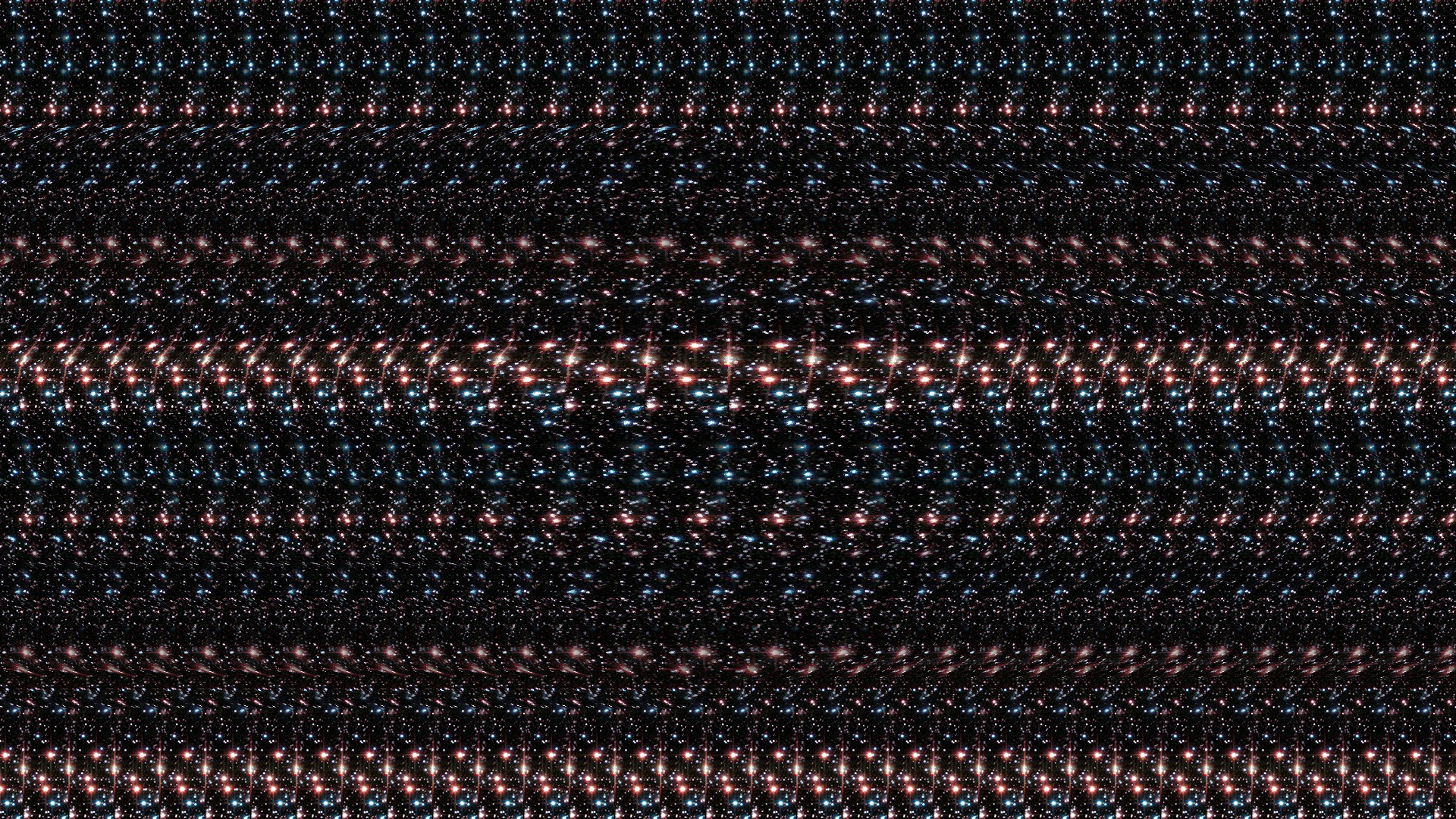# mathr / blog / #

## AutostereogramsWikipedia on Autostereograms doesn't exactly say how to construct them, so I drew some diagrams and scribbled some equations, and came up with this.

Given background distance and eye separation in inches, resolution in dots per inch, width in pixels, and count the number of vertical strips in the image background, compute the accomodation distance as follows:

accomodation = background * (1 - (width / count) / (separation * resolution))

This will be less than the background distance for positive eye separation (wall-eyed viewing) and greater for negative eye separation (cross-eyed viewing).

Then compute a depth value for each pixel, with the far plane at background inches from the camera. Ray marching a distance field is one way to do this, see Syntopia's blog for details. The scene should be between the camera and the far plane. Sharp depth discontinuities are disturbing, so position it as close to the far plane as possible.

The next step is converting the depth to a horizontal offset at the accomodation plane, using similar triangles:

delta = (depth - accomodation) * separation / depth;

Then compute the normalized texture coordinate increment that matches that offset:

increment[i] = 1 / (delta * resolution)

The i here is the horizontal index of the pixel, you need the whole scanline at a time if you want to center the texture instead of aligning it to an image edge. Now we have the speed of texture coordinate change, we can integrate this to get the actual texture coordinate for each pixel:

double sum = 0;
for (int i = 0; i < width; ++i) {
sum += increment[i];
coordinate[i] = sum;
}

and then do the texture lookup, rebasing it to the center of the image (twice % because negatives behave weird in C):

int u = floor((coordinate[i] - coordinate[width / 2]) * texture_width);
u %= texture_width;  u += texture_width;  u %= texture_width;
int v = j;
v %= texture_height; v += texture_height; v %= texture_height;
pixel[j][i] = texture[v][u];

Image above uses eye separation = -3 (cross-eyed), background distance = 12, 1920x1080 at 100dpi, count 32, the scene is a power 8 Mandelbulb copy-pasted from Fragmentarium, the texture is a slice of a NASA starfield image made seamless in GIMP.